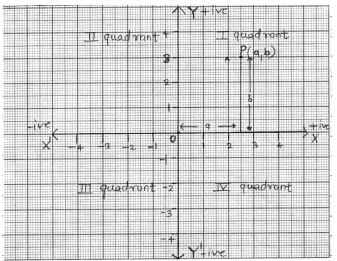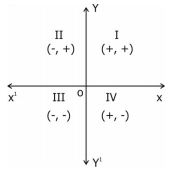### Coordinate Geometry - Revision Notes

CBSE Class 09  Mathematics

Revision Notes
CHAPTER – 3
COORDINATE GEOMETRY

1. Cartesian System
2. Plotting a Point in the Plane with given Coordinates

Coordinate Geometry : The branch of mathematics in which geometric problems are solved through algebra by using the coordinate system is known as coordinate geometry.

Coordinate System

Coordinate axes: The position of a point in a plane is determined with reference to two fixed mutually perpendicular lines, called the coordinate axes.In this system, position of a point is described by ordered pair of two numbers.

Quadrants: The coordinate axes divide the plane into four parts which are known as quadrants.

Ordered pair : A pair of numbers a and b listed in a specific order with 'a' at the first place and 'b' at the second place is called an ordered pair (a,b)

Note that $\left(a,b\right)\ne \left(b,a\right)$
Thus (2,3) is one ordered pair and (3,2) is another ordered pair.
In given figure O is called origin.
The horizontal line
XOX' is called the $x$-axis.
The vertical line YOY' is called the $y$-axis.
P(a,b) be any point in the plane. 'a' the first number denotes the distance of point from
$y$-axis and 'b' the second number denotes the distance of point from $x$-axis.
a - X - coordinate | abscissa of P.
b - Y - coordinate | ordinate of P.
The point of intersection of the coordinate axes is called the origin.
The coordinates of origin are (0,0)
Every point on the x-axis is at a distance o unit from the $x$-axis. So its ordinate is 0.
Every point on the y-axis is at a distance of unit from the $y$-axis. So, its abscissa is 0.Note : Any point lying on $x$- axis or $y$- axis does not lie in any quadrant.
The sign of coordinates (x, y) of a point in various quadrant are as given below:

 Quadrant Coordinates x y I + + II - + III - - IV + -